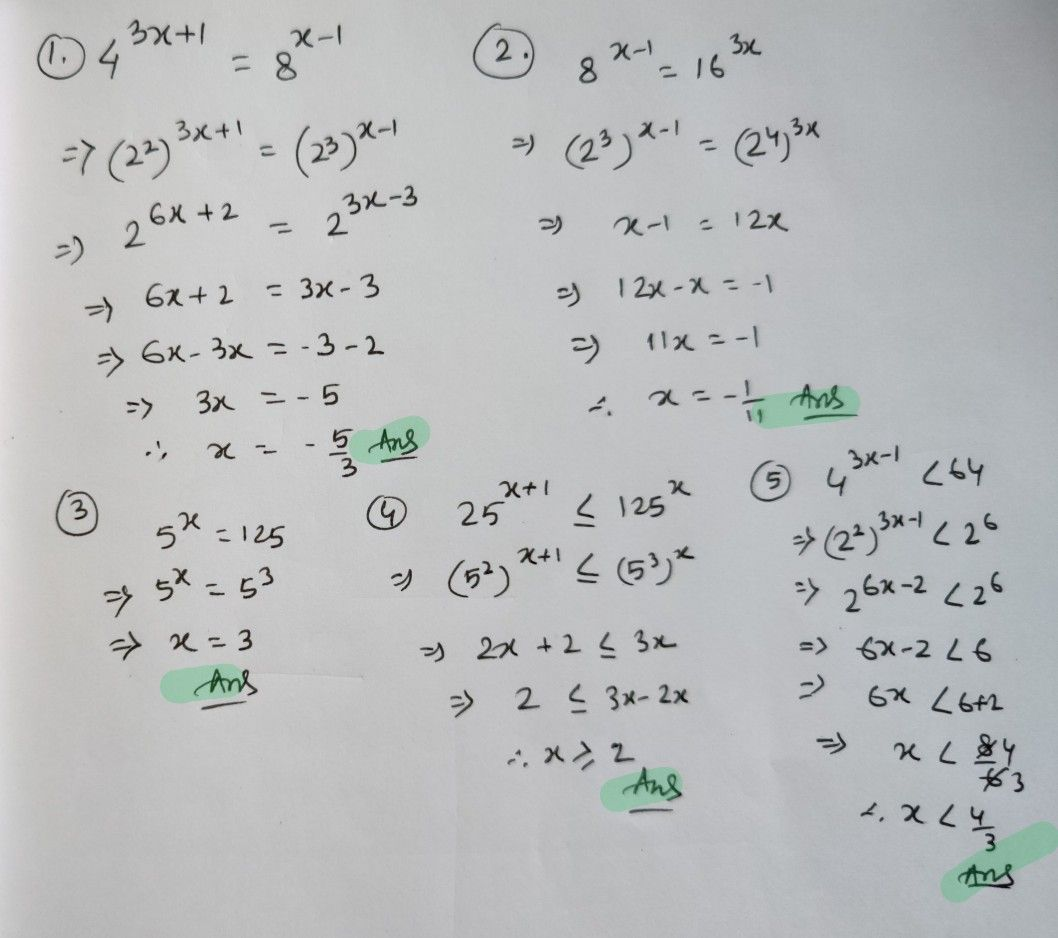Symbol
Problem$Di\pi ecH0ns$ Solve the following. Write your solution on the space provided. $1.$ $4^{3x+1}$ $1$ $=8^{x-1}$ $2.8^{x-1}=16^{3x}$ $3.5^{x}=125$ $4.25^{x+1}\leq 125^{x}$ $5.4$ $3x-1$ $<64$
10th-13th grade
Other
Search count: 111
Question content
Please help me with these. Thank youu I really need helpp
SolutionQanda teacher - RaiKindly Evaluate the Answer ?? if you're satisfied.Student
You're the best! Thank youu!